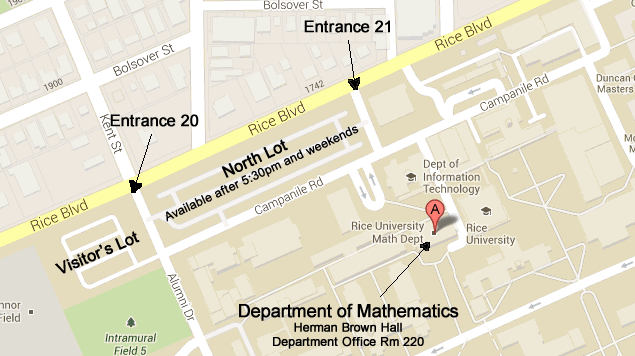## Differential geometry and geometric analysis

### Faculty

• Gregory Chambers

Metric geometry, and geometric analysis.

• Zhiyong Gao

Differential geometry.

• Robert Hardt (Emeritus)

Geometric measure theory, partial differential equations, continuum mechanics.

• F. Reese Harvey (Emeritus)

Several complex variables, partial differential equations, differential geometry.

• Chris Leininger

Geometry, topology, group theory, and dynamics in low dimension.

• Christos Mantoulidis

Differential geometry, Partial differential equations, Mathematical general relativity, Geometric measure theory, and Geometric flows.

• Jo Nelson

Symplectic and contact topology and geometry

Teichmüller theory, minimal surfaces, harmonic maps.

### InstructorsLarge Map

Tel (713) 348-4829